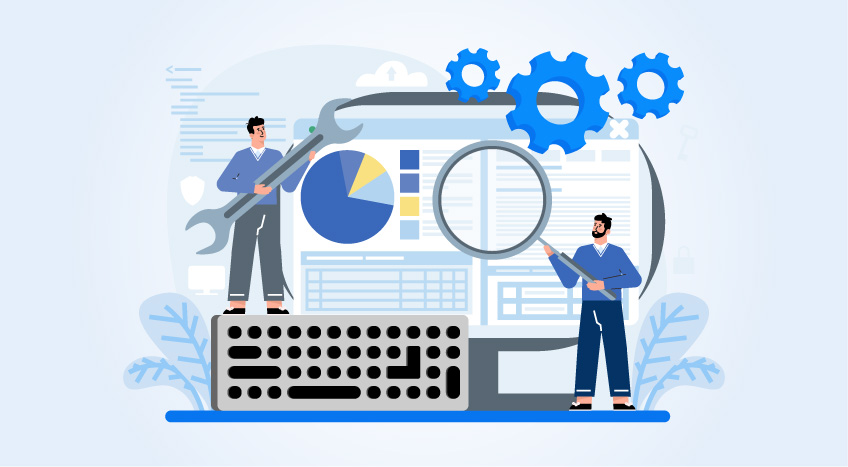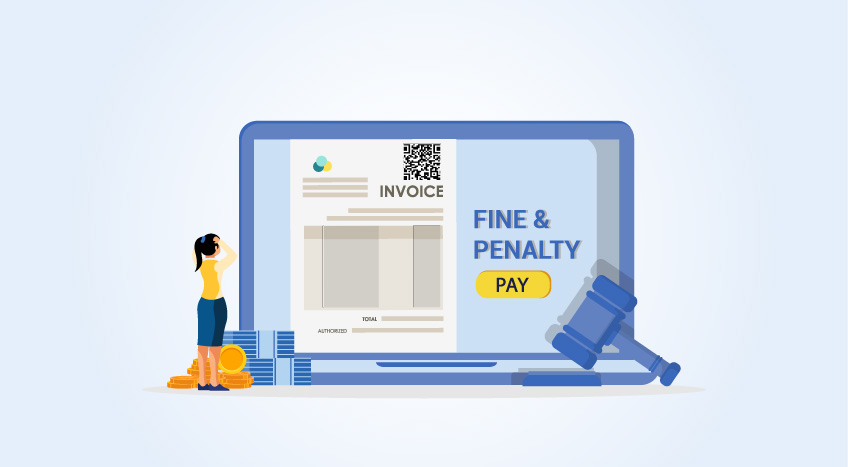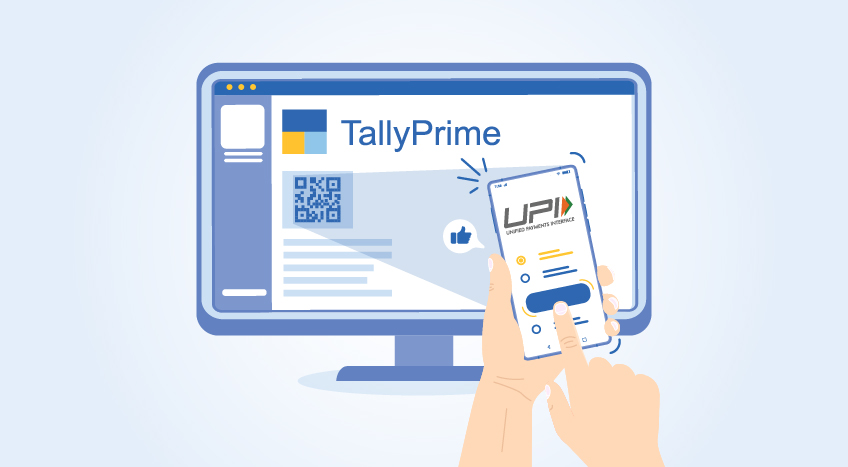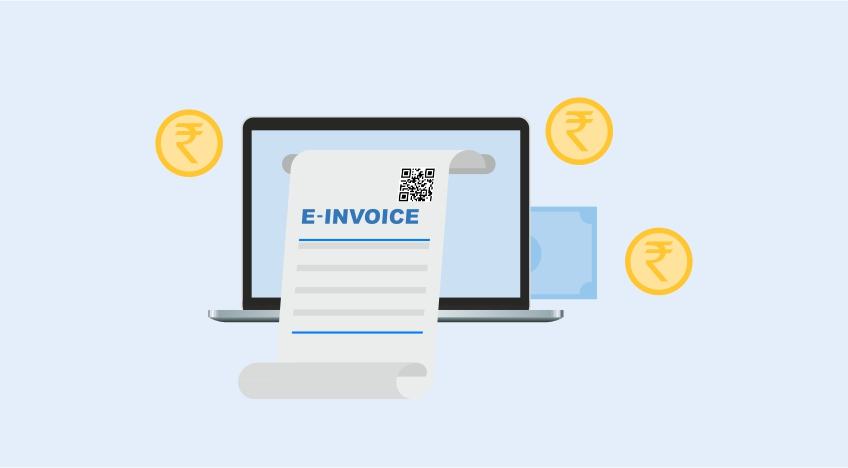# Difference Between Amortization and Depreciation

##### Tally Solutions|Updated on: June 29, 2022

At the end of every financial year, it is essential to calculate the company's asset value for the essential annual reports and tax purposes. There are two ways to calculate this value; depreciation and amortization. So, when a company buys an asset such as real estate or other assets, the difference between the two methods should be understood. Amortization is the method that is used to decrease the cost of the asset over time, while depreciation is the loss in value of the asset over time. This understanding helps in better understanding the financial implications of the purchase and saving time, effort, and money. The two calculations are important to calculate the tax liabilities and deductions over the asset's life.

## Similarities between amortization vs. depreciation

There are similarities between amortization and depreciation.

No cash: The expenses that are calculated for both amortization and depreciation are cashless. There is no actual cash expense that is made over the years for these amounts

Reporting: Both amortization and depreciation are stated as a reduction in fixed assets on the financial reports

Write off: Impairment or write off is when the book value of the assets are depreciated, causing a decrease in the amortization vs. depreciation balance

## Meaning of amortization

We often think of a company's assets as the real estate, plants, machinery, and equipment that is acquired by it. However, an asset can also be intangible such as customer lists, trade names, copyrights, patents, trademarks, franchisees, proprietary assets, bonds issued to raise capital, and employee relations or human capital. These intangible assets add significant value to a company. However, they can also lose value over time, and this loss in value is recorded on the financial statements through amortization.

The amortization value is usually calculated through the straight-line depreciation method, which means that the value that is recorded remains the same throughout the assets' useful life. This straight-line method of calculation is also used in accounting. Intangible assets, unlike tangible ones, do not have any salvage or resale values at the end of their usable life. Amortization also deals with the change in the value of intangible investments related to capital investments.

## Meaning of depreciation

Depreciation is a term used to expense the gradual decrease in value of a physical asset throughout its useful life. These tangible or fixed assets include real estate property, buildings, plants, machinery, equipment, vehicles, furniture, and other tangible items that the company owns.

The value of an item when it is brand new and after a period of use, sees a gradual reduction based on the period it has been used for. Tangible assets also have a residual value after the end of their useful life. The difference between this residual value and the cost of the asset is the depreciation. It is accounted for as a tax credit by the business through the asset’s useful life period.

An example could be a piece of machinery bought by a company. It is used for many years until it wears out beyond the point of repair or becomes obsolete. It may then be sold for scrap value. The loss of value that the machinery suffers through the years is depreciation. If the asset is a vehicle, the depreciation value is calculated at an accelerated speed at the beginning of the period as it loses most of its value in the first few years.

## Different ways to calculate depreciation

There are different standard methods of calculating the depreciation of an asset.

• Straight-line or linear method: This is a straightforward method where the loss in value is evenly distributed over a period of time. It is useful for items whose value declines steadily over time
• Declining or aggressive balance: This is a method of calculating depreciation at an unequal rate throughout the life of the asset. The decrease in value is accelerated in the earlier years and then becomes less aggressive over time. This is commonly used for items that become obsolete very quickly, such as computers, cell phones, and vehicles
• Double-declining balance: This is also a calculation method that calculates a higher decline in value early on and then a lower depreciation decrease
• Units of production: Instead of the value of the asset, this calculation uses the units that the asset produces over its lifetime rather than the value over the years of use
• Sum of years digits: The residual cost, useful life, and cost of the asset are all factored into this calculation method

## Amortization vs. depreciation – key differences

Definition: The definitions clearly illustrate the difference between amortization and depreciation. Depreciation calculates and refers to the loss of value of a tangible fixed asset over time. Depreciation can be applied to assets such as property, machinery, buildings, plants, equipment, and vehicles that the company owns. Amortization, by its definition, refers to the falling value of intangible assets over time. Amortization applies to intangible assets such as intellectual property, patents, trademarks, and other similar assets.

Formulae: The formulae that are used for the calculation of depreciation and amortization factor the fact that a tangible asset has some salvage or scrap value even at the end of its useful life. An intangible asset does not have this value and therefore, the formula for amortization does not include salvage value. The formulas show the difference between amortization and depreciation.

Annual Depreciation = (Cost of Tangible Asset-Salvage Value)/Useful Life

Annual Amortization= (Cost of Intangible Asset)/Useful Life

Materiality: There is no difference in the materiality of amortization and depreciation as they both calculate and account for the loss of value of assets over a period of time and record them as expenses on the company’s account statements.

Applicability: Applicability is a key difference between amortization and depreciation. Depreciation only applies to the tangible assets of the company. Amortization is applicable to the intangible assets of the company.

Implementation: Depreciation varies for different assets. Some assets depreciate at a steady rate over the years and are ideal for the straight-line method (SLM) of calculation. Other assets have an accelerated depreciation in the early years and then depreciate slowly. Amortization is most often calculated using the straight-line method.

Residual value: As seen in the formulae that are used for their calculation, tangible assets have a residual salvage value while intangible assets do not.

Recorded as expenses: Both depreciation and amortization are recorded as expenses on the company's financial statements and are often used as tax credits.

Journal entry: Both depreciation and amortization expenses are recorded as debits. Accumulated depreciation and amortization are recorded as credits.

Purpose: Depreciation is used to spread out the cost of the asset over the time period of its useful life. Amortization is used to capitalize the cost of the asset over a period of time. Depreciation is most commonly used for assets and is useful for gaining the company tax benefits.

Calculation example: Depreciation using the straight-line method

A company purchases a piece of equipment. Its useful life is expected to be around five years. The residual value at the end of the five years is expected to be 10% of the original purchase price.

Annual Depreciation = (Cost of Tangible Asset-Salvage Value)/Useful Life

Asset cost: \$ 10,000

Residual value after five years: \$ 10,000 x 10% = \$ 1,000

The difference between the asset cost and the residual cost = \$ 10,000 - \$ 1,000 = \$ 9,000

Annual depreciation = \$ 9,000 / 5 = \$ 1,800

Calculation example: Amortization

A company has been granted a maintenance contract for \$10 million which will be recovered over ten years.

Annual Amortization= (Cost of Intangible Asset)/Useful Life

Annual amortization = 10/10

Annual amortization cost = \$ 1 million

## Easily managing fixed assets

Both amortization and depreciation are ways to account for and spread the cost of an asset over the period of its useful life. The calculation of amortization and depreciation are both essential to record them as expenses on the financial statements and also for taxation purposes. However, they are applicable to different types of assets. Depreciation is calculated for tangible assets, and amortization is for intangible assets. Both amortization and depreciation are capital investments. But the accounting standards are different for depreciation and amortization. Take the hard work out of calculating depreciation and amortization for your business by using an intelligent accounting software solution TallyPrime. It keeps track of the depreciation calculation method and calculates it appropriately over the asset's useful life. TallyPime has a complete fixed assets analysis module that helps you minutely control the way you account for your fixed assets. It gives you all the details of the fixed assets that the company has carried into the current year and the ones that have been acquired or disposed of. It gives you a group level and individual level reporting on the fixed assets that the company holds.

Language

#### Latest Blogs